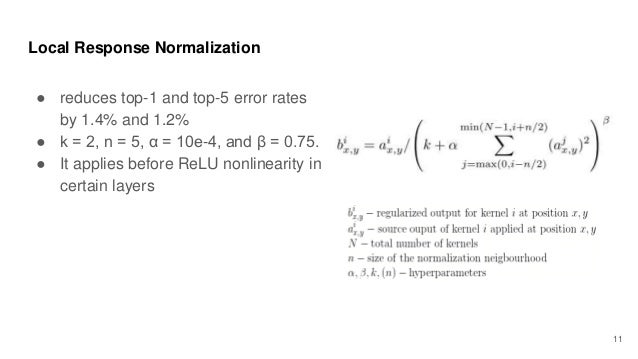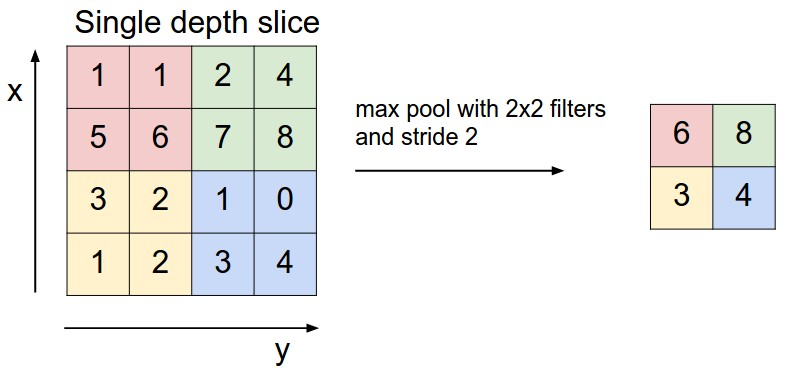This blog is mainly based on the paper “ImageNet Classification with Deep Convolutional Neural Networks”, in addition to the information obtained from fast.ai and Standford CS231N.

According to Serena Yeung (PhD at Stanford University, Co-lecturer of CS231N Convolutional Neural Networks for Visual Recognition) in her Lecture 5 (4:28 min), AlexNet in 2012 was the one of the deep learning architecture that sparked the whole use of convolutional neural networks more widely. AlexNet remarkably improved the top 1 and top 5 error rate on ImageNet Large-Scale Visual Recognition Challenge (ILSVRC) on 2010 and 2012 data sets than the previous state-of-the art results.

## What is ImageNet Large-Scale Visual Recognition Challenge (ILSVRC)?

ImageNet holds approximately 22,000 categories of 15 million images for visual object recognition research. With huge time and efforts invested in the project, ImageNet photos tend to be clean and training set images are labeled clearly for users.

The Challenge/Competition is based on a particular set from this database and is held every year since 2010. The purpose of the Challenge is to “evaluate algorithms for object detection and image classification at large scale.” Each ILSVRC is given with around 1,000 images per each category and 1,000 categories in a training set, 50,000 images in a validation set, and 150,000 images in a test set. For more information, the details are outlined on this place.

## Main characteristics of AlexNet

• Used deep eight layers, composed of 5 convolutional layers and 3 fully-connected layers.
• Had ~60 million parameters.
• Reduced Top 5 error rate by ~9% on ILSVRC-2010 test set.
• Found that an individual convolutional layer has an important role in the architecture/model because if a middle layer is removed, loss was worse about 2% for the top 1 error rate.
• Used dropouts to control overfitting.
• Structured as:

5 convolutional layers:

1. Convolutional layer

Activation layer (ReLU)

MaxPool

2. Convolutional layer

Activation layer (ReLU)

MaxPool

3. Convolutional layer

Activation layer (ReLU)

4. Convolutional layer

Activation layer (ReLU)

5. Convolutional layer

Activation layer (ReLU)

MaxPool

3 fully-connected layers:

Dropout

1. Linear

ReLU

Dropout

2. Linear

ReLU

3. Linear (Final classification layer)

## What is convolutional neural networks (CNN) and why does this CNN matter?

CNN uses a filter/kernel (for example, 3x3 image filter) to the input (e.g. image), passing the result to the next layer. The visualisation of the image filter/kernel is demonstrated well on this webpage. To a simpleton like me, CNN seems to be looking at an image through different types of a looking glass so that you can perceive the image from different perspectives to take in its diverse information.

There are three important numbers to see how CNN inputs and filters produce outputs.

1. input size: input size is usually height x width x RGB channels (e.g. 227 x 227 x 3).
2. filter size: filter size is based on the number of filters x height x width (e.g. 96 x 11 x 11).
3. stride: stride is how far the filter gets moved each time. Stride of 2 means it moves two steps to the right side.

Example of input and filter: The example below shows input size of 5 x 5 and filter size of 2 x 2 with stride of 1.To learn information from millions of images in thousands of categories, an architecture/model with a large learning capacity is required, generally made up of varying depth and breadth of layers. CNN tends to make mostly correct assumptions about the nature of images and has relatively fewer connections and parameters/weights compared to standard feedforward neural networks. This makes CNN more easier to train on the given data set.

However, CNN was expensive to apply in large scale to high resolution images. Thanks to (relatively) modern GPUs (then, the authors used two GTX580 3GB to train the data set between five to six days) and a highly optimised implementation of 2d convolutional layers (hereafter, conv layers), deep CNN became possible.

## How was the data processed and prepared?

The authors highlighted two pre-processing. First, they downsampled the images to a fixed resolution of 256 x 256. Also, they subtracted the mean activity over the training set from each pixel.

## What are novel features of the architecture (i.e. AlexNet)?

### 1. Rectified Linear Units (ReLUs)

The architecture needs an non-linear function, which we call an activation function. In old days, sigmoid, tahn and arctan used to be used widely for an activation function, but in this paper, the authors found that ReLU solves the problem of saturation better than traditional activation functions and does not require normalisation, which makes CNN with ReLU learns several times faster than tahn function.

ReLU sounds quite scary, but it is basically max(0, x). Therefore, the graph looks like this:source: http://cs231n.github.io/assets/nn1/relu.jpeg

More information about the activation function is in this video Lecture 6 (4:46 min) or you can read through this blog as well. They compared different activation functions and identified strengths and weaknesses.

### 2. Local Response Normalisation

Though ReLU does not require normalisation as long as training samples provides a positive input, the following expression of local response normalisation helped the authors to reduce their top-5 error rate by 1.2%.source: the expression on page 4 in the paper and https://image.slidesharecdn.com/alexnet1-180319134337/95/alexnetimagenet-classification-with-deep-convolutional-neural-networks-11-638.jpg?cb=1521467270

Honestly, I read this expression three times and still don’t get it fully. Update: Let us assume we have 6 filters and these filters all look at the position x (height) and the position y (width) of the input. Our goal is to normalise the particular value at this position with the 2nd (i-th) filter. To do so, we will divide this particular value by the sum of the squared values from its adjacent filters. In this example, if we assume n as 6 (n is a hyperparameter representing adjacent filters) and N as 5, j would be between 0 (max(0, 2-6/2)) and 4 (min(4, 2+6/2)). This means the value at the position x and y at the 2nd filter would be divided by the sum of the squared values at the same position between 0-th and 4-th filters.

• This has been added to To-come-back-to-understand-it-further list.

### 3. Overlapping pooling

Traditionally, stride and the height/width of a pooling unit was same, for example, stride was 2 and the pooling unit has the size of 2 x 2. However, in this paper, the authors used overlapping pooling by adopting stride lesser than the height/width of a pooling unit, which helped the reduction of Top-5 error rate ~0.3 %.

### 4. Overall architecture

Summary of AlexNet modelsource: Figure 2 in the paper and https://www.researchgate.net/profile/Jaime_Gallego2/publication/318168077/figure/fig1/AS:578190894927872@1514862859810/AlexNet-CNN-architecture-layers.png

By looking at the model above,

• the first layer represents an input layer;
• the second layer to the sixth layer are conv layers; and
• the last three layers are fully-connected layers.

To track the size of layers, this expression is helpful:

size of image/input through filters = (height/width of image/input - height/width of filter)/stride + 1

So the following example can be calculated as:

(4 - 2) / 2 + 1 = 2source: http://cs231n.github.io/assets/cnn/maxpool.jpeg

Overall, the size of output through filters, for example 96 different filters using the previous filter example, would be:

size of output through filters = the number of filters * size of image/input through filters * size of image/input through filters

Therefore, the final output size will be: 96 x 2 x 2

Applying the aforementioned expression to Summary of AlexNet model, the first layer (input layer) size should have been 227 x 227, not 224 * 224 (typo in the paper).

Pytorch implementation of the part of AlexNet (copied from Pytorch github) is as follow (comments of the layer size are made by me):

## How did the authors control overfitting?

### 1. Data augmentation

The authors artificially enlarged the dataset through five image translations and five horizontal reflection (total 10 different augmentation options per image) and merged the predictions made by the network’s softmax layer (i.e. the last layer) on the ten patches.

To change intensities of the RGB in training images, the authors did Principal Component Analysis (PCA) on the set of RGB pixel values for the training set and added the principal components discovered (with certain proportions) to each RGB image pixel.

### 2. Dropout

Dropout sets the output of the applied hidden layer to zero with a desired probability. This is to simulate combining the predictions of many different models efficiently (noise/diversity helps to generalise better to reduce test errors) because training each model takes several days. Dropouts were applied to first two fully-connected layers with the probability 0.5.

## How did the authors train the architecture?

The model was trained with stochastic gradient descent with momentum of 0.9, weight decay of 0.0005, and batch size of 128 images. These are important hyperparameters because the rule for training the weights of the model is as follow:source: https://i.stack.imgur.com/RfFkY.png

My attempt to interpret this expression is that the next weight is based on the current weight and the next momentum. This next momentum value is updated through 90% of the current momentum value, subtracting 1) 0.05% of learning rate multiplied by the current weight; and 2) learning rate multiplied by the average over the current iteration index’s batch of the derivative (of the objective) with respect to w, evaluated at the current weight.

I am still trying to understand the last part of this expression.

• This has been added to To-come-back-to-understand-it-further list.

Weights were initialised with a zero mean Gaussian distribution and standard deviation of 0.01. Biases (constant 1) were added in the second, fourth, fifth conv layers and the first two fully-connected layers. Learning rate was starting from 0.01 with a division by 10 through the training iterations.

## What were the results?

In summary, AlexNet achieved the reduction of Top-1 error rate around 8% and Top-5 error rate around 9% on ILSVRC-2010. On the 2012 Challenge, the authors pre-trained the model on the ImageNet 2011 Fall dataset release and reduced the error rate down to 15.3% (previously 17% on the 2010 test set).

## Lessons learnt

This was my first attempt to read through and understand the deep learning paper over a few days. AlexNet paper had several concepts that I had to explore further, such as a size of layer, an advantage of ReLU over tahn, a visualisation of a conv layer through filters, and the implementation of AlexNet in Pytorch. There were the two math expressions that I had a difficult time to take in, but I decided to come back to them later rather than being stuck behind stumbling blocks.